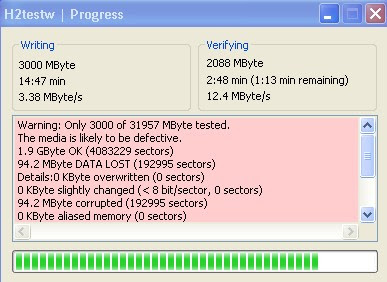When we buy some storage devices such as hard disks, USB flash drives, memory cards, etc., the first thing is to see if there is such a large capacity, but every time we look at the capacity, everyone is a little disappointed because of the purchase It's less than the capacity you want to buy, and some are even less. So some people wonder if the merchant is selling fakes. Here I will tell you why the storage devices you buy are always less than the actual capacity.For example : 32G U disk, but only shows 29.2GB

Everyone sees the first reflection here is not feeling that this is a fake? Actually not, they are just different algorithms, and there are so many real capacities.

In our normal algorithm, 1GB is equal to 1000MB, 1MB is equal to 1000KB, and 1KB is equal to 1000byte.
The system algorithm we use is 1GB equal to 1024MB, 1MB equals 1024KB, and 1KB equals 1024byte.

So, for example, the hard disk we see is 223.57, and the real capacity is equal to 223.57GB multiplied by 1.024 times 1.024 times 1.024.

You can calculate it yourself to see if it is equal to this value.

When we buy some storage devices such as hard disks, USB flash drives, memory cards, etc., the first thing is to see if there is such a large capacity, but every time we look at the capacity, everyone is a little disappointed because of the purchase It's less than the capacity you want to buy, and some are even less. So some people wonder if the merchant is selling fakes. Here I will tell you why the storage devices you buy are always less than the actual capacity.For example : 32G U disk, but only shows 29.2GB

Everyone sees the first reflection here is not feeling that this is a fake? Actually not, they are just different algorithms, and there are so many real capacities.

In our normal algorithm, 1GB is equal to 1000MB, 1MB is equal to 1000KB, and 1KB is equal to 1000byte.
The system algorithm we use is 1GB equal to 1024MB, 1MB equals 1024KB, and 1KB equals 1024byte.

So, for example, the hard disk we see is 223.57, and the real capacity is equal to 223.57GB multiplied by 1.024 times 1.024 times 1.024.

You can calculate it yourself to see if it is equal to this value.